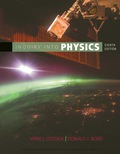# Determine the range of wavelengths in the UV radiation band.### Inquiry into Physics

8th Edition
Ostdiek
Publisher: Cengage
ISBN: 9781337515863

#### Solutions

Chapter
Section### Inquiry into Physics

8th Edition
Ostdiek
Publisher: Cengage
ISBN: 9781337515863
Chapter 8, Problem 7P
Textbook Problem
6 views

## Determine the range of wavelengths in the UV radiation band.

To determine

The range of wavelengths in the UV radiation band.

### Explanation of Solution

Given information:

The range of wavelengths in the UV radiation band.

Formula used:

The frequency of the wave can be calculated as:

c = λ f.

Calculation:

Frequency range of UV is 7.5×1014Hz to 1×1018Hz, then the frequency of the wave is:

c=λfλ=cfwhen f=7.5×1014Hz then λ=3× 1087

### Still sussing out bartleby?

Check out a sample textbook solution.

See a sample solution

#### The Solution to Your Study Problems

Bartleby provides explanations to thousands of textbook problems written by our experts, many with advanced degrees!

Get Started

Find more solutions based on key concepts
Organic nutrients include all of the following except. a. minerals b. fat c. carbohydrates d. protein

Nutrition: Concepts and Controversies - Standalone book (MindTap Course List)

In exergonic reactions, the energy is: a.used. b.stored. c.released. d.lost.

Chemistry for Today: General, Organic, and Biochemistry

50. Name each compound:

Chemistry In Focus

Astronomers detect a distant meteoroid moving along a straight line that, if extended, would pass at a distance...

Physics for Scientists and Engineers, Technology Update (No access codes included)

Meteors in showers were once part of which type of celestial object?

Foundations of Astronomy (MindTap Course List)